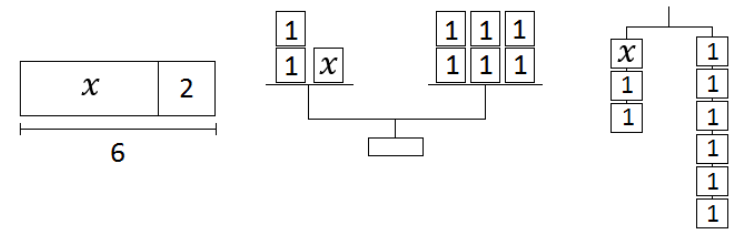# Equations and Inequalities

Lesson 4

Math

Unit 6

Lesson 4 of 14

## Objective

Solve one-step equations with addition and subtraction.

## Common Core Standards

### Core Standards

• 6.EE.B.6 — Use variables to represent numbers and write expressions when solving a real-world or mathematical problem; understand that a variable can represent an unknown number, or, depending on the purpose at hand, any number in a specified set.
• 6.EE.B.7 — Solve real-world and mathematical problems by writing and solving equations of the form x + p = q and px = q for cases in which p, q and x are all nonnegative rational numbers.

• 5.NF.A.1
• 6.NS.B.3

## Criteria for Success

1. Understand that in the process of solving for a variable, whatever is done to one side of the equation must also be done to the other side in order to maintain the balance.
2. Use models and diagrams to solve for a variable.
3. Solve one-step addition and subtraction equations algebraically.
4. Write and solve one-step addition and subtraction problems for real-world contexts.

## Tips for Teachers

Students first solve equations by using diagrams; they then generalize their actions to solve equations algebraically without diagrams. Though some students may find they can mentally solve these equations, encourage students to organize and show their thinking algebraically. This will support them later as the equations become more and more complex.

Fishtank Plus

Unlock features to optimize your prep time, plan engaging lessons, and monitor student progress.

## Anchor Problems

### Problem 1

The following three diagrams represent the equation ${x+2=6}$. Explain how you can use each one to find the value of $x$.How can you solve the equation ${m+3.6=9.2}$ without using a diagram?

### Problem 2

Draw a tape diagram to represent the equation ${d-5=7}$; then use it to find the value of $d$.

How can you solve the equation ${p-{3\over4}=8}$ without using a diagram?

#### References

EngageNY Mathematics Grade 6 Mathematics > Module 4 > Topic G > Lesson 26Exercise 2

Grade 6 Mathematics > Module 4 > Topic G > Lesson 26 of the New York State Common Core Mathematics Curriculum from EngageNY and Great Minds. © 2015 Great Minds. Licensed by EngageNY of the New York State Education Department under the CC BY-NC-SA 3.0 US license. Accessed Dec. 2, 2016, 5:15 p.m..

Modified by Fishtank Learning, Inc.

### Problem 3

At the grocery store, Martin uses some coupons and saves $5.75 off his total bill. His new bill comes to$21.36. Let $x$ represent the amount of Martin’s grocery bill before using the coupons.

Write and solve an equation to find the amount of Martin’s bill before using the coupons.

## Problem Set

The following resources include problems and activities aligned to the objective of the lesson that can be used to create your own problem set.

• Include problems with procedural practice in solving addition and subtraction problems.
• Include problems where students write an equation from a context (addition or subtraction only) and solve the equation.
• Include error analysis problems of mistakes made in solving equations.
• Include problems with procedural practice in solving addition and subtraction problems.
• Include problems where students write an equation from a context (addition or subtraction only) and solve the equation.
• Include error analysis problems of mistakes made in solving equations.

### Problem 1

Solve the equations. Show or explain your thinking.

a.   ${w+1.5=8.75}$

b.   ${z-12=9}$

### Problem 2

A large bowl holds ${5{2\over3}}$ cups of punch. Some more punch is added to the bowl to make a total of 10 cups of punch. Let $c$ represent the number of cups of punch that are added to the bowl.

Write and solve an equation to find the number of cups of punch that are added to the bowl.

Lesson 3

Lesson 5

## Lesson Map

Topic A: Reasoning About and Solving Equations

Topic B: Reasoning About and Solving Inequalities

Topic C: Representing and Analyzing Quantitative Relationships